# Wolfram 语言™

## 原理和概念 计算智能平台的基础核心思想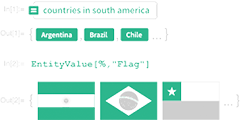### 基于知识的编程

• 迄今为止最大的算法网络集合
• 涵盖了三十年多来在 Mathematica 中开发的高级算法
• 世界上最大的可计算知识的集合
• 不断精选在 Wolfram|Alpha 中使用的上千个领域的数据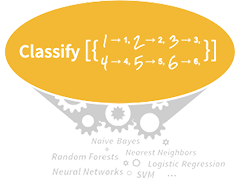### 元算法和超级函数

Wolfram 语言的理念是最大限度的自动化，因此程序员只需专注于界定他们想要做的，语言可以自动分析出解决方法。

• 拥有可供自动算法选择的上千种独创的元算法
• 向专业人员提供细节调控功能；其他人员则可利用自动操作功能
• 计算、演示、连接、界面等的自动化
• 最小化代码的长度和复杂性### 所有方面的完美统和

• 跨越所有领域的即时互用性
• 最大化编程架构的灵活性
• 最大化可预测性和可学习性
• 编码的可读性和可理解性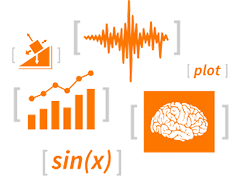### 所有内容都是表达式

Wolfram 语言用符号表达式表示所有数据、公式、代码、图形、文档、界面等，使得编程的灵活性和能力达到一个新的水平。

• 增量编程：任意片段的程序都可以立即运行
• 可立刻在系统中表示任何格式的数据
• 代码中可包含任意对象，比如图像和文档等
• 程序可以立即操纵结构和内容### WDF：Wolfram 数据框架

• 对单位、日期和地理位置等的完美处理
• 对成千上万真实世界实体的标准表示
• 表示真实世界数据的可扩展的符号式结构框架
• 不断更新在 Wolfram|Alpha 中实际测试的知识库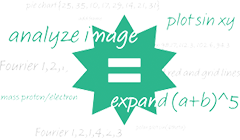### 自然语言理解（NLU）

• 无需编程知识便可开始使用 Wolfram 语言
• 用日常名称便捷指定真实世界的实体
• 广泛的自然语言理解（NLU）、在 Wolfram|Alpha 中的实际测试
• 在编程时，用 NLU 指定真实世界的对象和概念
• 在您编写的程序中加入对自然语言的理解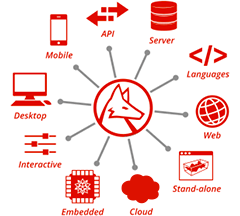### 通用部署

• 在云端或本地的畅通运行
• 对任何 Wolfram 语言程序快速创建网页 API
• 在软件或硬件系统中无缝嵌入 Wolfram 语言
• 用 Wolfram 语言符号式描述其自身部署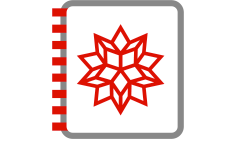### CDF：可计算文档格式

Wolram 语言的内置“笔记本”文档将可执行代码与文本、图形、界面等相混合。

• 创建一个含有编码、范例、说明等的单个文档
• 程序化创建功能齐全的报告和文档
• 快速创建由计算支持的交互式元素
• Wolfram 演示项目中涵盖了上万个各类范例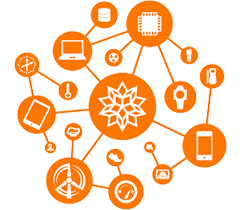### WSTP（Wolfram 符号化传输协定），Wolfram 设备连接项目等

Wolfram 语言中内置有与多种语言、服务、程序、格式和设备的连通功能。

• 符号表达式标准化与外部数据和程序的交互操作
• 通过 Wolfram Cloud 与外部进行无缝连接
• 在语言中直接处理与设备的实时交互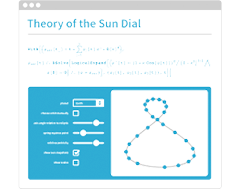### 一切都是交互式的

Wolfram 语言的原生环境有着完全的交互性，并可以让您快速运行任意一段代码。

• 快速试运行您编辑的所有内容
• 即刻生成视图并分析您的程序代码
• 无缝隙地进行增量或探索编程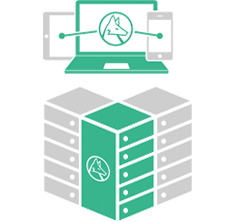### 完全的伸缩性

Wolfram 语言的大小可从单行程序到数百万行程序，并可用于单个用户以及大型公共部署。

• 用于交互使用和大型编程的便捷 IDE
• 创建 Wolfram 语言代码并可立即并行执行
• 年度单行竞赛展示语言表现力
• Wolfram|Alpha 包含超过 1500 万行的 Wolfram 语言代码库### 多范型融合语言

• 几乎所有的 Wolfram 语言都要比其他语言简洁
• 内置结构直接与概念相连接
• 大范围工业强度的函数编程
• 基于模式的符号编程
• 强大的理论基础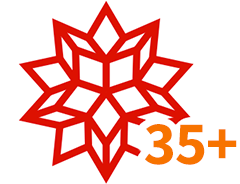### 三十多年的持续演变

• 持续三十年以上的设计审查过程
• 由 Stephen Wolfram 带领的长期团队